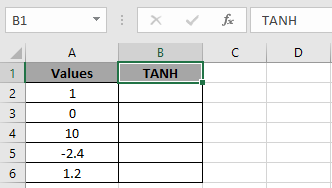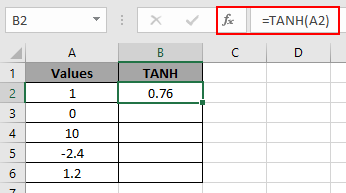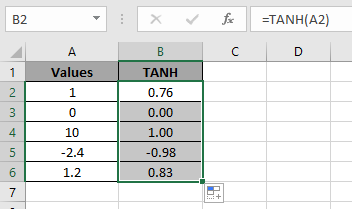# How to use the TANH Function in Excel

TANH function is built in excel function. Hyperbolic function of TANH defined using the exponential function.

TANH (x) = SINH (x) / COSH (x)
TANH (x) = (ex- e-x) / (ex+ e-x)

Where ex = exponential function, e = 2.71828

The TANH function is hyperbolic Tangent of the input number in radians. The function returns a number.
Syntax:

=TANH (number)

number : input number you want the hyperbolic Tangent of.

Let’s understand this function using it in an example.Here we have values and we need to find its hyperbolic Tangent value.

Use the formula:

=TANH(A2)

A2 : number given as cell reference.The value will calculated using the mathematical formulation stated :
TANH (x) = (e1- e-1) / (e1+ e-1)
Now copy the formula to other cells using the Ctrl + D shortcut key.Excel calculates the Hyperbolic Tangent of a number using the TANH function.

Notes:

1. The function returns the #VALUE! Error, if the argument to the function is non numeric.
2. The input number is the angle in radians.
3. If you want the result in degrees, multiply the result by 180/PI ().
4. If the argument provided is in degrees, first multiply the number in degrees by PI ()/180 to convert it into radians.

Hope you understood how to use TANH function and referring cell in Excel. Explore more articles on Excel mathematical and trigonometric functions here. Please feel free to state your query or feedback for the above article.

Popular Articles:

50 Excel Shortcuts to Increase Your Productivity

How to use the VLOOKUP Function in Excel

How to use the COUNTIF in Excel 2016

How to use the SUMIF Function in Excel

Terms and Conditions of use

The applications/code on this site are distributed as is and without warranties or liability. In no event shall the owner of the copyrights, or the authors of the applications/code be liable for any loss of profit, any problems or any damage resulting from the use or evaluation of the applications/code.